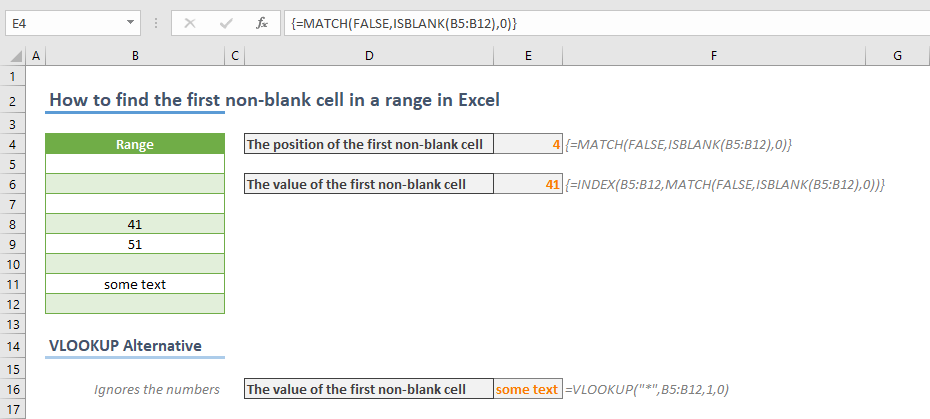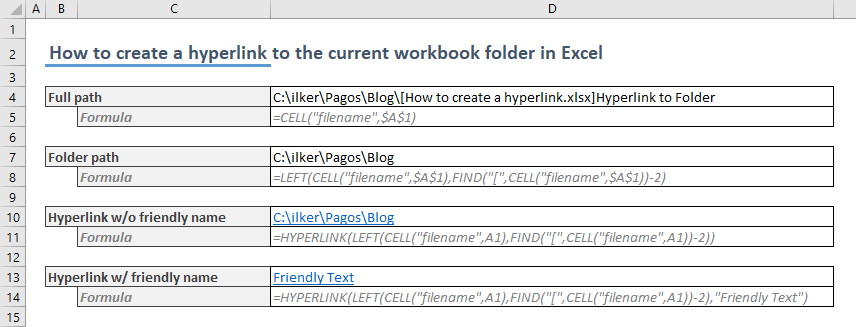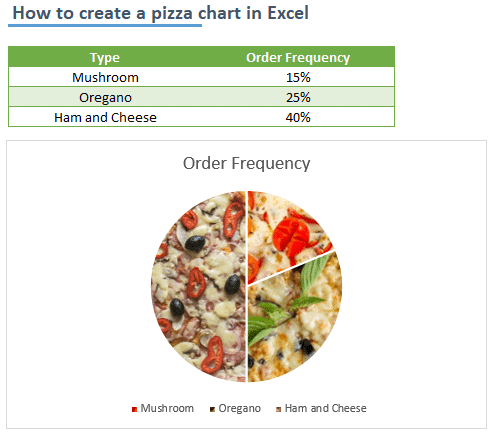## How to cut and paste transpose in Excel

A common problem when transposing data in Excel is that data is populated in their relative cells. This means that you may occasionally see messed up references when using the Paste function with transpose. In this article, we are going to show you how to cut and...## How to find the first non-blank cell in a range in Excel

If you frequently analyze data in Excel, you may come across empty cells before the actual values. Instead of removing these cells, you can get the position or even the value of the first non-blank cell with formulas. In this article, we are going to show you how to...## How to count the number of cells greater than the average in Excel

In this article, we are going to show you how to count the number of cells greater than the average in Excel. Download Workbook Formula =COUNTIFS(<numbers range>,”>“&AVERAGE(<numbers range>)) <numbers range>: The reference from the range of...## How to count the number of cells less than the average in Excel

In this article, we are going to show you how to count the number of cells that is less than the average of that data set in Excel. Download Workbook Formula =COUNTIFS(<numbers range>,”<“&AVERAGE(<numbers range>)) <numbers range>: The...## How to create a hyperlink to the current workbook folder in Excel

A hyperlink is not only for navigation between internet pages! Just like with websites, hyperlinks can be very useful in Excel too. You can even create hyperlinks to sheets or references in an Excel workbook as well. For example, you can generate a hyperlink to the...## How to create a pizza chart in Excel

Of course, there isn’t a chart type called a pizza chart in Excel. On the other hand, Excel has a support for inserting images into chart elements. With help of this cool feature, you can give your charts a fancy look. In this article, we are going to show you how to...